• 对现有的音频文件进行傅里叶分析，多个程序，MATLAB编程
• 汉宁窗傅里叶变换matlab代码Matlab音频过滤 为了充分了解设备的性能，还必须在频域中分析信号。 这正是频谱分析仪的工作。 但是，应该指出的是，随着数字技术的飞速发展，这种区别变得越来越模糊。 一些示波器可以...
• 使用Matlab 编写一段傅里叶变换代码，对你所采集的 wav 文件中的一段(1-2s)进行傅里叶变换，并显示频谱。 我使用的音频是琵琶D调Re，文件命名为test.wav。记得修改为自己的文件名哦。 代码如下： [xx,fs]=audioread...
本博客实验环境是Matlab2018，软件版本不同可能会有些出入，需要稍作修改。
使用Matlab 编写一段傅里叶变换代码，对你所采集的 wav 文件中的一段(1-2s)进行傅里叶变换，并显示频谱。
我使用的音频是琵琶D调Re，文件命名为test.wav。记得修改为自己的文件名哦。 代码如下：
[xx,fs]=audioread('test.wav');
x=xx(:,1);
N=length(x);
time=(0:N-1)/fs;
plot(x);
M=2048;
nfft=8192;
win=hanning(M);
freq=(0:nfft/2)*fs/nfft;
y=x(9001:9000+M);
y=y-mean(y);
Y=fft(y.*win,nfft);
figure(2)
subplot 211;plot(y);xlim([0 M]);
title('一帧信号波形');xlabel('样点');ylabel('幅值')
subplot 212;plot(freq,20*log10(abs(Y(1:nfft/2+1))));
grid;axis([0 max(freq) -60 55]);
title('频谱');xlabel('频率(Hz)');ylabel('幅值')


运行结果如下所示：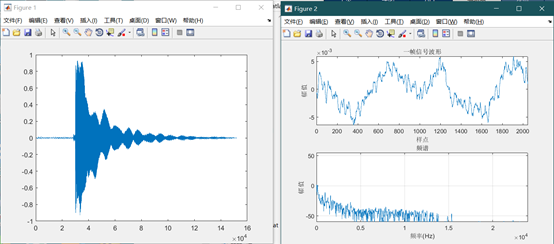展开全文• 利用 Matlab 编写一段傅里叶变换代码，对你所采集的 wav 文件中的一段(1-2s)进行傅里叶变换，并显示频谱，同时与 Audition 的频谱图进行对比。 代码： [xx,fs]=wavread('7.1.1000.wav');#7.1.1000.wav是进行傅里叶...
利用 Matlab 编写一段傅里叶变换代码，对你所采集的 wav 文件中的一段(1-2s)进行傅里叶变换，并显示频谱，同时与 Audition 的频谱图进行对比。 代码：
[xx,fs]=wavread('7.1.1000.wav');#7.1.1000.wav是进行傅里叶变换的文件
x=xx(:,1);
N=length(x); time=(0:N-1)/fs; plot(x);
M=2048;
nfft=8192;
win=hanning(M);
freq=(0:nfft/2)*fs/nfft;
y=x(9001:9000+M); y=y-mean(y); Y=fft(y.*win,nfft); figure(2)
subplot 211; plot(y); xlim([0 M]); title('一帧信号波形'); xlabel('样点'); ylabel('幅值')
subplot 212; plot(freq,20*log10(abs(Y(1:nfft/2+1)))); grid; axis([0 max(freq) -60 55]);
title('频谱'); xlabel('频率(Hz)'); ylabel('幅值')

结果：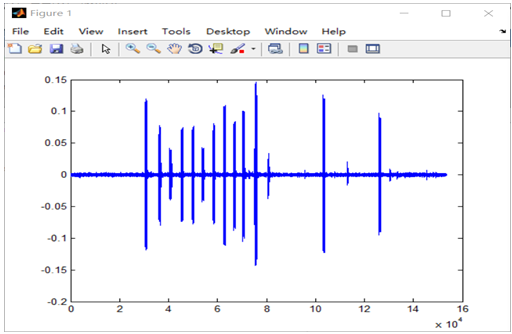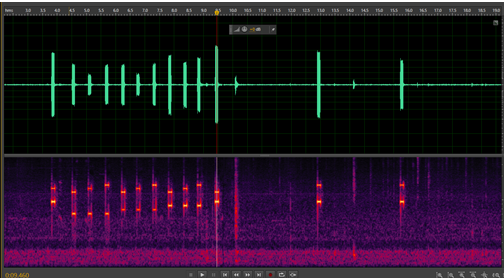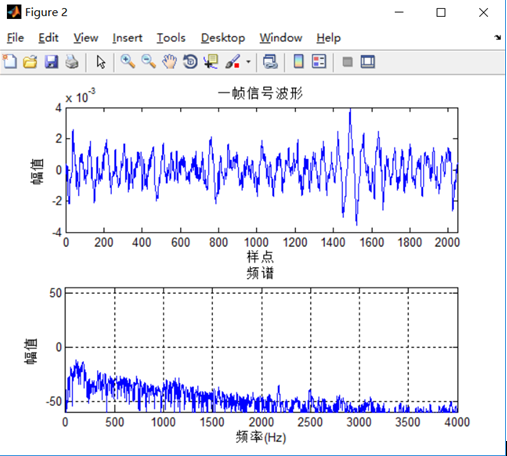展开全文• Matlab是一个在很多科学和工程领域都非常有用的数学工具。傅里叶变换在信号处理、物理、通信、地质学、天文学、...快速傅里叶变换是一种高效进行离散傅里叶变换的方法，并且存在很多种方法来完成快速傅里叶变换Matlab
Matlab是一个在很多科学和工程领域都非常有用的数学工具。傅里叶变换在信号处理、物理、通信、地质学、天文学、光学等很多领域都有应用。这个技术将一个函数或是一组数据从时域或是取样域变换到频域。这意味着，傅里叶变换可以展示一组时间序列数据的频率分量。离散傅里叶变换是将取样域的离散数据转化到频域。快速傅里叶变换是一种高效进行离散傅里叶变换的方法，并且存在很多种方法来完成快速傅里叶变换。Matlab 使用快速傅里叶变换来得到离散数据的频域分量。下面是一个在 Matlab 中如何用快速傅里叶变换来分析音频文件的例子。这个例子中的文件是记录在 A5上的音叉录音。这个展示了傅里叶变换如何进行和如何在 Matlab 中使用这项技术。

function test_20161214

Nsamps = length(y);
t = (1/Fs)*(1:Nsamps)          %Prepare time data for plot

%Do Fourier Transform
y_fft = abs(fft(y));            %Retain Magnitude
y_fft = y_fft(1:Nsamps/2);      %Discard Half of Points
f = Fs*(0:Nsamps/2-1)/Nsamps;   %Prepare freq data for plot

%Plot Sound File in Time Domain
figure
plot(t, y)
xlabel('Time (s)')
ylabel('Amplitude')
title('Tuning Fork A4 in Time Domain')

%Plot Sound File in Frequency Domain
figure
plot(f, y_fft)
xlim([0 1000])
xlabel('Frequency (Hz)')
ylabel('Amplitude')
title('Frequency Response of Tuning Fork A4')

快速傅里叶变换用 “fft” 函数执行。Matlab 没有 “dft” 函数因为快速傅里叶变换实际上就是计算的离散傅里叶变换。尽管快速傅里叶变换的角度在很多应用中非常中央，但是只有快速傅里叶变换的大小被保存了。“fft” 函数允许指定一个快速傅里叶变换的输出点数，但是在这个例子中，我们使用与输入点数一样数目的输出点数。在下一行，快速傅里叶变换的一半数据被舍弃了。为了这个例子的目的所以这样做了，但是在很多应用中，整个波谱都会用到（译者注：我认为这里舍弃一半的点是因为FFT是关于采样频率的一半对称的，所以只要看一半就可以了）。接下来的一行，将会用于横坐标的数据通过使用采样频率和时遇采样数量准备好了。这一步对于确定包含在音频文件的实际频率是很重要的。
接下来，原始数据在时域上被画了下来，快速傅里叶变换的数据也被画了出来。为了展示在峰值的频率上的更多详情，在这个画中，x轴被限定在了 [0,1000] 的范围中。注意，在大约440Hz处，频率响应有一个峰值，这个就是 “A5” 的频率。在其他频率也有一些很小东西，这些估计是音叉了。对于其他乐器比如吉他，在频率响应的峰值的整数倍上都可以看见谐波。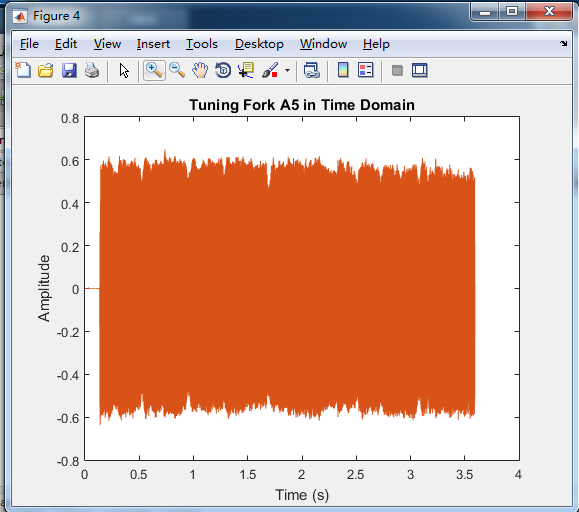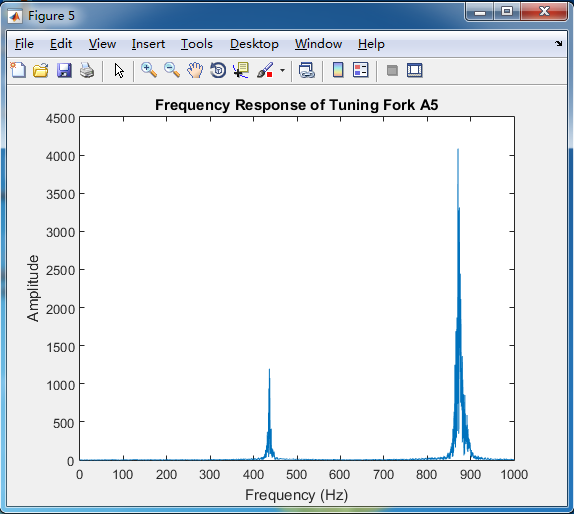傅里叶变换在很多不同的领域都是很有用的工具。二维的傅里叶变换也常常用在图像上。尝试一下上面的代码，看看你能不能得到一样的结果。
PS：有人问 FFT 结果的幅值问题。本质来说，FFT结果的幅值单位是什么并不重要，只要你在分析过程中，需要分析的两个幅值的单位是统一的就可以了。Matlab 的 FFT 最终结果的绝对值的确看上去并不好看（太大了），根据 Matlab 帮助文件，FFT 的最终结果还需要进行一下小调整再来使用比较好（上面的图未作调整），以下是我根据 Matlab 帮助文档编写的一个计算 FFT 并绘制 FFT 结果幅值图的函数，具体如何使用，请看函数内的注释说明。
function [ frequency,fft_result ] = fft_plot( data,Fs,varargin )
% Calculate or plot directly fft results of data.
%
% [ frequency,fft_result ] = fft_plot( data,Fs,'plot' )
%
% inputs:
%   (1) data: data used to analysis. one row -> one data
%   (2) Fs: sample frequency
%   (3) 'plot': veriable input. if there is not this input, fft results will not be
%   ploted
% output:
%   (1) freqeuncy: frequency corresponding to the fft results
%   (2) fft_result: fft results

if nargin<2
error('data and Fs must be given');
elseif nargin==2
for k=1:size(data,1)
size_data=size(data(k,:));
if size_data(1)~=1 && size_data(2)~=1
error('the length or the number of rows must be one.');
end
data(k,:)=data(k,:)-mean(data(k,:));
L=length(data(k,:));
NFFT=2^nextpow2(L);
fft_result_temp=fft(data(k,:),NFFT)/length(data(k,:));
fft_result(k,:)=fft_result_temp(k,1:NFFT/2+1);
frequency(k,:)=Fs/2*linspace(0,1,NFFT/2+1);
end
elseif nargin==3
figure;
title('FFT')
for k=1:size(data,1)
if strcmp(varargin,'plot')
size_data=size(data(k,:));
if size_data(1)~=1 && size_data(2)~=1
error('the length or the number of rows must be one.');
end
data(k,:)=data(k,:)-mean(data(k,:));
L=length(data(k,:));
NFFT=2^nextpow2(L);
fft_result_temp=fft(data(k,:),NFFT)/length(data(k,:));
frequency(k,:)=Fs/2*linspace(0,1,NFFT/2+1);
fft_result(k,:)=fft_result_temp(1:NFFT/2+1);
subplot(size(data,1),1,k);
plot(frequency(k,:),2*abs(fft_result(k,:)));
xlabel('Frequency')
ylabel('Amplitude')
else
error('variable input must be ''plot''');
end
end
elseif nargin>=3
error('Too much inputs')
end
end


不懂的可以加我的QQ群：522869126（语音信号处理） 欢迎你的

到来哦，看了博文给点脚印呗，谢谢啦~~


展开全文语音识别
• 这段代码实现的是MATLAB基于离散小波变换（DWT）的语音和音频信号的数字水印代码，有GUI几乎每句都有详细的注释，附带一个录音的小程序，其中加入了两种干扰，一是低通滤波，二是白噪声干扰。另外还附赠解释小波变换...
• 对一段音频信号进行短时傅里叶变换（或小波变换matlab源码），处理得到含频率和时间成分的语谱图（matlab+python源码）
• 本程序是短时傅里叶变换matlab程序，短时傅里叶变换是信号分析的重要手段，在视频，图像，音频信号中有着非常重要的作用。
• 在本教程中，我们将了解如何将这种思考方式作为傅立叶变换进行操作。 在进行生理测量时，通过在频域中操纵输入的时间序列数据（例如，滤除与“噪声”相关的频率范围）来增强信号至关重要。 友好的介绍（外部资源） ...
• 本文主要给定一小段音频，通过短时傅里叶变换和小波变换制作时频图。 0、准备工作 首先先准备音频，预先用ffmpeg切割一段时长为1s的音频音频的采样率为44100，但采样到的点数为46076个点，时长约为1.04s。   1...
本文主要给定一小段音频，通过短时傅里叶变换和小波变换制作时频图。
0、准备工作
首先先准备音频，预先用ffmpeg切割一段时长为1s的音频，音频的采样率为44100，但采样到的点数为46076个点，时长约为1.04s。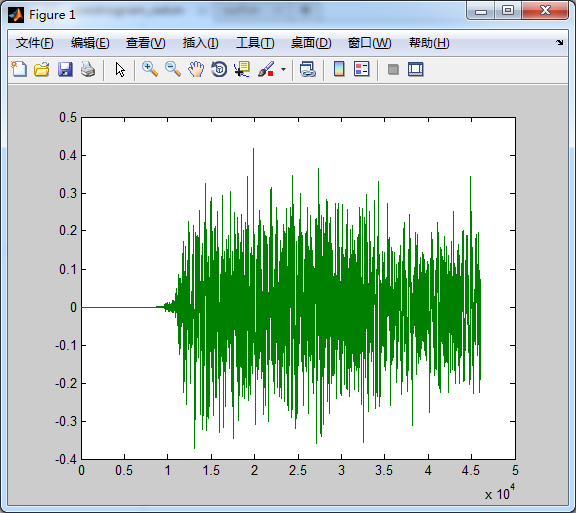1、短时傅里叶变换
首先，在matlab中，短时傅里叶变换的分析函数为spectrogram，其使用情况如下：

语法：

[S,F,T,P]=spectrogram(x,window,noverlap,nfft,fs)       [S,F,T,P]=spectrogram(x,window,noverlap,F,fs)         说明：当使用时无输出参数，会自动绘制频谱图；有输出参数，则会返回输入信号的短时傅里叶变换。当然也可                 以从函数的返回值S,F,T,P绘制频谱图，具体参见例子。         参数：                 x---输入信号的向量。默认情况下，即没有后续输入参数，x将被分成8段分别做变换处理，如果x不能被平   分成8段，则会做截断处理。默认情况下，其他参数的默认值为                 window---窗函数，默认为nfft长度的海明窗Hamming                 noverlap---每一段的重叠样本数，默认值是在各段之间产生50%的重叠                 nfft---做FFT变换的长度，默认为256和大于每段长度的最小2次幂之间的最大值。另外，此参数除了使用一 个常量外，还可以指定一个频率向量F                 fs---采样频率，默认值归一化频率。
Window---窗函数，如果window为一个整数，x将被分成window段，每段使用Hamming窗函数加窗。如果   window是一个向量，x将被分成length(window)段，每一段使用window向量指定的窗函数加窗。所    以如果想获取specgram函数的功能，只需指定一个256长度的Hann窗。
Noverlap---各段之间重叠的采样点数。它必须为一个小于window或length(window)的整数。其意思为两个相邻窗不是尾接着头的，而是两个窗有交集，有重叠的部分。                 Nfft---计算离散傅里叶变换的点数。它需要为标量。                 Fs---采样频率Hz，如果指定为[]，默认为1Hz。                 S---输入信号x的短时傅里叶变换。它的每一列包含一个短期局部时间的频率成分估计，时间沿列增加，频率沿行增加。                 如果x是长度为Nx的复信号，则S为nfft行k列的复矩阵，其中k取决于window，                 如果window为一个标量，则k = fix((Nx-noverlap)/(window-noverlap))                 如果window为向量，则k = fix((Nx-noverlap)/(length(window)-noverlap))                 对于实信号x，如果nfft为偶数，则S的行数为(nfft/2+1)，如果nfft为奇数，则行数为(nfft+1)/2，列数同上。                 F---在输入变量中使用F频率向量，函数会使用Goertzel方法计算在F指定的频率处计算频谱图。指定的频率被四舍五入到与信号分辨率相关的最近的DFT容器(bin)中。而在其他的使用nfft语法中，短时傅里叶变换方法将被使用。对于返回值中的F向量，为四舍五入的频率，其长度等于S的行数。                 T---频谱图计算的时刻点，其长度等于上面定义的k，值为所分各段的中点。                 P---能量谱密度PSD(Power Spectral Density)，对于实信号，P是各段PSD的单边周期估计；对于复信号，当指定F频率向量时，P为双边PSD。
MATLAB程序：

	[Au, Fs]=audioread('C:\Users\CDQ\Desktop\output.mp3');   % Fs 采样率 44100
[B, F, T, P] = spectrogram(Au(:,1),1024,512,1024,Fs);   % B是F大小行T大小列的频率峰值，P是对应的能量谱密度
figure
imagesc(T,F,C);
set(gca,'YDir','normal')
colorbar;
xlabel('时间 t/s');
ylabel('频率 f/Hz');
title('短时傅里叶时频图');


运行结果：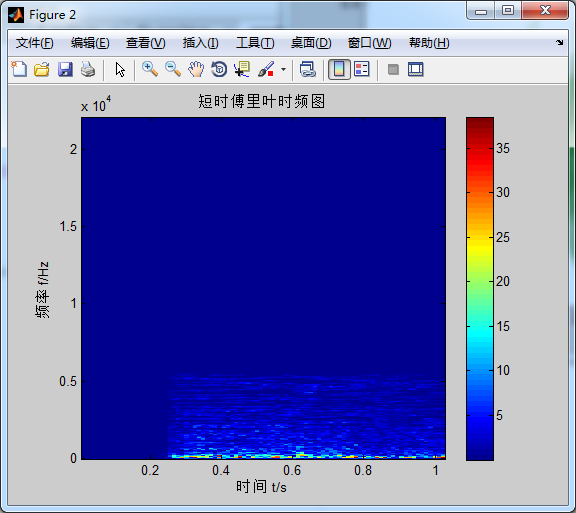2、小波变换
首先，在matlab中，小波变换的分析函数为cwt，其使用情况如下：
cwt函数功能：实现一维连续小波变换的函数。         cwt函数语法格式：

COEFS=cwt(S, SCALES, 'wname')

COEFS=cwt(S, SCALES, 'wname', 'plot')
COEFS=cwt(S, SCALES, 'wname', 'PLOTMODE')

COEFS=cwt(S, SCALES, 'wname', 'PLOTMODE', XLIM)

使用说明：cwt为一维小波变换的函数。

格式 COEFS=cwt(S, SCALES, 'wname') 采用'wname'小波，在正、实尺度SCALES下计算向量一维小波系数。

格式 COEFS=cwt(S, SCALES, 'wname', 'plot') 除了计算小波系数外，还加以图形显示。

格式 COEFS=cwt(S, SCALES, 'wname', 'PLOTMODE') 计算并画出连续小波变换的系数，并使用PLOTMODE对图形着色。

格式 COEFS=cwt(S, SCALES, 'wname', 'plot') 相当于 格式 COEFS=cwt(S, SCALES, 'wname', 'PLOTMODE') 中的语法 COEFS=cwt(S, SCALES, 'wname', 'absglb')

格式 COEFS=cwt(S, SCALES, 'wname', 'PLOTMODE', XLIM) 能够计算并画出连续小波变换的系数。系数使用PLOTMODE和XLIM进行着色。其中：XLIM=[x1,x2]，并且有如下关系：1<=x1<=x2<=length(S)。

MODE值含义：

'lvl' scale-by-scale着色模式

'glb' 考虑所有尺度的着色模式

'abslvl'或'lvlabs' 使用系数绝对值的scale-by-scale着色模式

'absglb'或'glbabs' 使用系数绝对值并考虑所有尺度的着色模式

COEFS行的大小等于SCALES尺度的长度，COEFS列的大小等于信号S的长度。

MATLAB程序：

	wavename='cmor3-3';
totalscal=1024;
Fc=centfrq(wavename); % 小波的中心频率  测得Fc = 3
c=2*Fc*totalscal;    % 测得c = 1536
scals=c./(1:totalscal);
f=scal2frq(scals,wavename,1/fs); % 将尺度转换为频率   频率在0-500Hz取1024个点
coefs = cwt(Au(:,1),scals,wavename); % 求连续小波系数
t=0:1/fs:size(Au(:,1))/fs;
figure
imagesc(t,f,abs(coefs));
set(gca,'YDir','normal')
colorbar;
xlabel('时间 t/s');
ylabel('频率 f/Hz');
itle('小波时频图');
运行结果：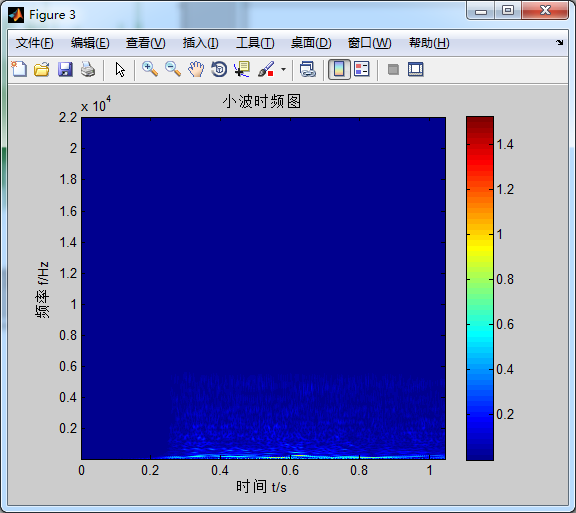展开全文• 傅里叶变换matlab代码Zaf-Python Zafar的Python音频功能，用于音频信号分析。 档案： ：具有音频功能的Python模块。 ：Jupyter笔记本，并提供一些示例。 ：用于示例的音频文件。 也可以看看： ： Matlab中Zafar的...
• 所谓盲水印，是指人感知不到的水印，包括看不到或听不见（没错，数字盲水印也能够用于音频）。其主要应用于音像作品、数字图书等，目的是，在不破坏原始作品的情况下，实现版权的防护与追踪。 添加数字盲水印的方法...隐藏水印
• 汉宁窗傅里叶变换matlab代码光谱 用于绘制音频频谱的MATLAB脚本 描述 频谱 读取音频文件，进行播放并实时绘制频谱。 频谱的样式取决于所选择的refreshFig函数。 要更改refreshFig功能，只需将refreshFig.m替换为...
• 摘要：录制一段音频，分别采用matlab，python两种方式，对其作短时傅里叶变换（STFT），最终得到期望的语谱图。 一、前言 基础概念： 什么是傅里叶变换傅里叶的基本定义与性质在这里就不作赘述了，文章主要想...python 信号处理 小波分析
• Matlab短时傅里叶变换和小波变换的时频分析 简介 本文主要给定一小段音频，通过短时傅里叶变换和小波变换制作时频图。音频的采样率为44100， 短时傅里叶变换matlab中，短时傅里叶变换的分析函数为...
• 然后对音频进行快速傅里叶变换fft(y,N)，N取32768，画出信号的频谱特性，加深对频谱特性的理解。（3）根据频谱，反演时域特性，画出时域波形。寻找幅值最大的两个频率，此频率除以fft点数在乘以采样频率就是信号的...
• 一、函数调用 二、函数源码 function d=stftms(x,win,nfft,inc) if length(win)==1 % 判断有否设置窗... % 进行傅里叶变换 d(:,c) = t(1:(1+nfft/2)); % 取1到1+nfft/2之间的谱值 c = c+1; % 改变帧数，求取下一帧 end;
• 从《法证先锋4》的一段...至于具体的操作，剧中原话是这样的：MFCC（梅尔频率倒谱系数）除了可以像剧中那样用来做声纹鉴定，更广泛的用途是进行音乐风格分类、音乐检索和语音识别等。那么MFCC到底是什么东西？MFC...
• ## 【音频处理】离散傅里叶变换

万次阅读 多人点赞 2018-06-12 18:03:03
最近复现音乐驱动舞蹈的文章《Dancing-to-Music Character Animation》，用到了与傅里叶变换很相似的称为常Q变换的方法去分割音乐，所以对傅里叶变换做了一个小了解，本文不深入各种乱糟糟的理论，比如什么蝶形算法...
• ## 从傅里叶变换到加窗傅里叶变换

万次阅读 多人点赞 2017-12-10 14:00:49信号与系统 信号处理
• matlab实现傅里叶变换代码STFT_Python 在MATLAB中的短时傅立叶变换（STFT）效果是通过Python代码实现的（利用Python代码实现的matlab中的短时傅里叶变换效果） 使用“ pip install -r requirements.txt”安装从属库...
• 这是一组解决人类语音处理基本任务的脚本： 频谱分析频谱控制均衡频谱偏移（上/下） 平方阈值它显示了时频二元性，如何正确... 它包含一组用于测试脚本的 wav 文件，听产生的音频很有趣：如金属声音或男性\女性效果。
• 这篇博文中使用的模拟信号为上篇博文：【 MATLAB 】使用 MATLAB 实现模拟信号的近似及其连续傅里叶变换 中使用的模拟信号： 为了研究在频域数量上的采样效果，对该信号使用两种不同的采样频率采样。 a. 在 fs =...
• Gabor变换也叫窗口傅里叶变换 对于给定的常数a,b,称 为在窗口函数g下的Gabor变换。 窗口函数一般是具有紧支集或者速降为零的函数，常用的窗口函数有三个： 对于已知的分段线性函数如下，求其Gabor变换的...信号处理
• DFT的matlab源代码频谱图 提取音频信号（wav）并将其转换为频谱图。 用Go编程语言编写。 安装 git克隆 例子 python3 sine_wav.py && ../../xigh/spectrogram/spectrogram -hamming -hideavg -bins = 256 sine.wav ...
• ## 【音频处理】短时傅里叶变换

万次阅读 多人点赞 2018-06-13 17:18:13
上一篇博客讲了离散傅里叶变换，里面的实例是对整个信号进行计算，虽然理论上有N点傅里叶变换(本博客就不区分FFT和DFT了，因为它俩就是一个东东，只不过复杂度不同)，但是我个人理解是这个N点是信号前面连续的N个...
• 从 GUI 可以导入任意数据或音频信号。 接下来我们可以在时域和频域中绘制它们。 我们还可以可视化该信号的短时傅立叶变换
• 傅里叶变换matlab代码薄膜晶体管 STFT（短时傅立叶变换），ISTFT（逆-短时傅立叶变换），用于音频，麦克风输入 提供25％，50％的重叠STFTCraft.io。 笔记 git clone --recursive ...要构建测试代码，您需要克隆--...
• 使用加窗傅立叶变换研究低通音频滤波器和信号处理 该项目使用多种类型的窗口傅立叶变换来研究时频域中的音乐样本。 我特别研究了这种变换的调谐，以及海森堡不确定性对时间和频率分辨率的限制。 我还执行泛音过滤并......

# matlab音频进行傅里叶变换matlab 订阅